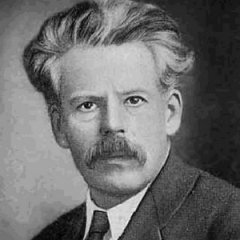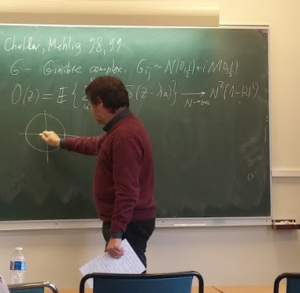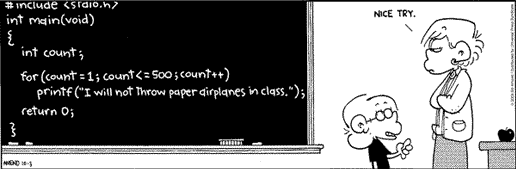Press "Enter" to skip to content

# Month: December 2017Evgeny Evgenievich Slutsky (1880 – 1948).

This tiny back to basics post is devoted to a couple of bits of Probability and Statistics.

The central limit theorem cannot hold in probability. Let ${{(X_n)}_{n\geq1}}$ be iid real random variables with zero mean and unit variance. The central limit theorem (CLT) states that as ${n\rightarrow\infty}$,

$Z_n=\frac{X_1+\cdots+X_n}{\sqrt{n}} \overset{\text{law}}{\longrightarrow}\mathcal{N}(0,1).$

A frequently asked question by good students is to know if one can replace the convergence in law by the (stronger) convergence in probability. The answer is negative, and in particular the convergence cannot hold almost surely or in ${L^p}$. Let us examine why. Recall that the convergence in probability is stable by linear combinations and by subsequence extraction.

We proceed by contradiction. Suppose that ${Z_n\rightarrow Z_\infty}$ in probability. Then necessarily ${Z_\infty\sim\mathcal{N}(0,1)}$. Now, on the one hand, ${Z_{2n}-Z_n\rightarrow0}$ in probability. On the other hand,

$Z_{2n}-Z_n =\frac{1-\sqrt{2}}{\sqrt{2}}Z_n+\frac{X_{n+1}\cdots+X_{2n}}{\sqrt{2n}} =\frac{1-\sqrt{2}}{\sqrt{2}}Z_n+\frac{1}{\sqrt{2}}Z_n’.$

But ${Z_n’}$ is an independent copy of ${Z_n}$. Thus the CLT used twice gives ${Z_{2n}-Z_n\overset{\text{law}}{\longrightarrow}\mathcal{N}(0,\sigma^2)}$ with ${\sigma^2=(1-\sqrt{2})^2/2+1/2=2-\sqrt{2}\neq0}$, hence the contradiction.

Alternative proof. Set ${S_n=X_1+\cdots+X_n}$, and observe that

$\frac{S_{2n}-S_n}{\sqrt{n}}=\sqrt{2}Z_{2n}-Z_n.$

Now, if the CLT was in probability, the right hand side would converge in probability to ${\sqrt{2}Z_\infty-Z_\infty}$ which follows the law ${\mathcal{N}(0,(\sqrt{2}-1)^2)}$. On the other hand, since ${S_{2n}-S_n}$ has the law of ${S_n}$, by the CLT, the left hand side converges in law towards ${Z_\infty\sim\mathcal{N}(0,1)}$, hence the contradiction. This “reversed” proof was kindly suggested by Michel Ledoux.

Intermezzo: Slutsky lemma. The Slutsky lemma asserts that if

$X_n\overset{\text{law}}{\longrightarrow} X \quad\text{and}\quad Y_n\overset{\text{law}}{\longrightarrow} c$

with ${c}$ constant, then

$(X_n,Y_n)\overset{\text{law}}{\longrightarrow}(X,c),$

and in particular, ${f(X_n,Y_n)\overset{\text{law}}{\longrightarrow} f(X,c)}$ for every continuous ${f}$.

Let us prove it. Since ${Y_n\overset{\text{law}}{\longrightarrow} c}$ and ${c}$ is constant, we have ${Y_n\rightarrow c}$ in probability, and since for all ${t\in\mathbb{R}}$, the function ${y\mapsto \mathrm{e}^{ity}}$ is uniformly continuous on ${\mathbb{R}}$, we have that for all ${s,t\in\mathbb{R}}$ and all ${\varepsilon>0}$, there exists ${\eta>0}$ such that for large enough ${n}$,

$\begin{array}{rcl} |\mathbb{E}(\mathrm{e}^{isX_n+itY_n})-\mathbb{E}(\mathrm{e}^{isX_n+itc})| &\leq&\mathbb{E}(|\mathrm{e}^{itY_n}-\mathrm{e}^{itc}|\mathbf{1}_{|Y_n-c|\leq\eta})+2\mathbb{P}(|Y_n-c|>\eta)\\ &\leq& \varepsilon+2\varepsilon. \end{array}$

Alternatively we can use the Lipschitz property instead of the uniform continuity:

$\begin{array}{rcl} |\mathbb{E}(\mathrm{e}^{isX_n+itY_n})-\mathbb{E}(\mathrm{e}^{isX_n+itc})| &\leq&\mathbb{E}(\left|\mathrm{e}^{itY_n}-\mathrm{e}^{itc}\right|\mathbf{1}_{|Y_n-c|\leq\eta})+2\mathbb{P}(|Y_n-c|>\eta)\\ &\leq& |t|\eta+2\varepsilon. \end{array}$

On the other hand, since ${X_n\overset{\text{law}}{\longrightarrow}X}$, we have, for all ${s,t\in\mathbb{R}}$, as ${n\rightarrow\infty}$,

$\mathbb{E}(\mathrm{e}^{isX_n+itc})=\mathrm{e}^{itc}\mathbb{E}(\mathrm{e}^{isX_n}) \longrightarrow \mathrm{e}^{itc}\mathbb{E}(\mathrm{e}^{isX}) =\mathbb{E}(\mathrm{e}^{isX+itc}).$

The delta-method. Bizarrely this basic result, very useful in Statistics, appears to be unknown to many young probabilists. Suppose that as ${n\rightarrow\infty}$,

$a_n(Z_n-b_n)\overset{\text{law}}{\longrightarrow}L,$

where ${{(Z_n)}_{n\geq1}}$ is a sequence of real random variables, ${L}$ a probability distribution, and ${{(a_n)}_{n\geq1}}$ and ${{(b_n)}_{n\geq1}}$ deterministic sequences such that ${a_n\rightarrow\infty}$ and ${b_n\rightarrow b}$. Then for any ${\mathcal{C}^1}$ function ${f:\mathbb{R}\rightarrow\mathbb{R}}$ such that ${f'(b)\neq0}$, we have

$\frac{a_n}{f'(b)}(f(Z_n)-f(b_n))\overset{\text{law}}{\longrightarrow}L.$

The typical usage in Statistics is for the fluctuations of estimators say for ${a_n(Z_n-b_n)=\sqrt{n}(\widehat{\theta}_n-\theta)}$. Note that the rate in ${n}$ and the fluctuation law are not modified! Let us give a proof. By a Taylor formula or here the mean value theorem,

$f(Z_n)-f(b_n)=f'(W_n)(Z_n-b_n)$

where ${W_n}$ is a random variable lying between ${b_n}$ and ${Z_n}$. Since ${a_n\rightarrow\infty}$, the Slutsky lemma gives ${Z_n-b_n\rightarrow0}$ in law, and thus in probability since the limit is deterministic. As a consequence ${W_n-b_n\rightarrow0}$ in probability and thus ${W_n\rightarrow b}$ in probability. The continuity of ${f’}$ at point ${b}$ provides ${f'(W_n)\rightarrow f'(b)}$ in probability, hence ${f'(W_n)/f'(b)\rightarrow1}$ in probability, and again by Slutsky lemma,

$\frac{a_n}{f'(b)}(f(Z_n)-f(b_n)) =\frac{f'(W_n)}{f'(b)}a_n(Z_n-b_n) \overset{\text{law}}{\longrightarrow}L.$

If ${f'(b)=0}$ then one has to use a higher order Taylor formula, and the rate and fluctuation will be deformed by a power. Namely, suppose that ${f^{(1)}(b)=\cdots=f^{(r-1)}(b)=0}$ while ${f^{(r)}(b)\neq0}$, then, denoting ${L_r}$ the push forward of ${L}$ by ${x\mapsto x^r}$, we get

$\frac{a_n^rr!}{f^{(r)}(b)}(f(Z_n)-f(b_n)) \overset{\text{law}}{\longrightarrow}L_r.$

The delta-method can be of course generalized to sequences of random vectors, etc.

Last Updated on 2018-01-26

J’ai fini par remiser mon Origine Tuxedo au profit d’un vélo moins fragile, mieux adapté à mon nouveau trajet. Voici donc ma nouvelle bicyclette. Un poids relativement faible, de gros pneus, des freins à disques hydrauliques, 2×10 vitesses classiques, des porte-bagages. Un régal.P.S. : je ne crève plus depuis mon passage aux pneus Schwalbe Marathon Plus Tour.

Last Updated on 2020-03-11Yan Fyodorov lors du MEGA à l’IHP. Sur le tableau la subsistance du N après le passage à la limite 🙂

J’étais vendredi à l’Institut Henri-Poincaré  pour les exposés mensuel du MEGA (Matrices Et Graphes Aléatoires). Saviez-vous que cet institut doit son existence notamment aux efforts de Émile Borel – mathématicien et homme politique, figure sans doute très inspirante pour Cédric Villani – ainsi qu’aux moyens de la Fondation Rockefeller et de Edmond de Rothschild ?

Le cours didactique du matin était donné par Laurent Ménard, et portait sur la combinatoire analytique à la Philippe Flajolet. Il s’agit typiquement d’obtenir des formules de comptage notamment asymptotiques en utilisant l’arsenal de l’analyse complexe (intégrales de contour, méthode du col, …) à partir d’identités combinatoires fonctionnelles sur les fonctions génératrices. D’après Laurent une excellente référence est le livre Analytic combinatorics.

Le premier exposé de l’après-midi était donné par l’énergique Yan Fyodorov et portait sur l’article arXiv:1710.04699 concernant des formules explicites pour des statistiques liées aux vecteurs propres pour les modèles matriciels gaussiens de Ginibre réel et complexe. Le second était donné par Alice Guionnet et portait sur l’étude de modèles de gaz discrets avec beta variable, en liaison par exemple avec l’article arXiv:1705.05527. Dans les deux cas, la virtuosité et l’arsenal techniques sont impressionnants. Ces deux orateurs sont des sommités mondiales.

Il semble que dans le domaine des matrices aléatoires, la plupart des questions simples abordables ont déjà été explorées. Doit-on s’attendre dans le futur à des vagues de travaux simplificateurs ? C’est ce qu’on peut souhaiter au sujet. Certains pensent que pour survivre au temps, les mathématiques ont besoin d’être simples et profondes et que cela résulte d’une lente digestion collective. Au delà de ce domaine, il est frappant de constater la place grandissante de la sophistication technique dans les mathématiques actuelles. On se prend à douter parfois.

En tout cas, ces trois exposés étaient passionnant et enthousiasmants !Auto-apprentissage ? On évoque souvent ces entrepreneurs du numérique autodidactes qui ont appris à programmer seuls, qui ont abandonné leurs études, et qui ont créé des entreprises à succès. Les exemples ne manquent pas : Bill Gates (Microsoft), Xavier Niel (Free), Mark Zuckerberg (Facebook), etc. La programmation se prête effectivement particulièrement bien à l’auto-apprentissage, et cette idée est d’ailleurs au cœur de la très médiatisée École 42. Au delà de la programmation, l’auto-apprentissage est un moteur puissant pour ceux qui sont à la fois doués et passionnés, mais encore faut-il avoir le goût de l’effort, ce qui n’est pas simple en ces temps d’enfants rois. En tout les cas, il n’a jamais été aussi facile d’auto-apprendre et Internet joue un rôle fantastique en la matière en donnant accès à beaucoup de ressources de qualité.

L’apprentissage n’est pas que ludique et passe aussi par des aspects exigeants ou rébarbatifs qui rebutent les esprits peu enclins à faire des efforts. Bien que le discours autour de l’auto-apprentissage puisse être parfois démagogique, il véhicule malgré tout un message positif, un message de réussite possible pour ceux à qui le système éducatif traditionnel ne réussit pas assez. Mais faut-il vraiment détruire les écoles et universités traditionnelles pour construire des écoles 42 ? Il n’y a pas d’opposition à faire entre éducation traditionnelle et auto-apprentissage car nous avons tous besoin de multiples modes d’apprentissage. Le cas des chercheurs est intéressant. Ce sont souvent au départ des élèves doués, passionnés, et persévérants, qui ont réussi dans le système éducatif traditionnel, tout en auto-apprenant tout au long de leur parcours. Ils ont souvent croisé des enseignants, des livres, ou des ressources Internet qui les ont séduits. Enfin leur métier même de chercheur fait intervenir une dose massive d’auto-apprentissage. C’est aussi le cas, dans une certaine mesure, pour beaucoup d’ingénieurs.

Last Updated on 2020-03-07

Syntax · Style · .